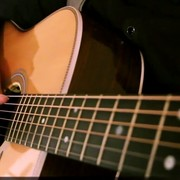{meta: 原调: 1=D} {meta: 选调: 1=D} {meta: Capo=0} {comment: 前奏} [Gsus4] [Dadd9] [Gsus4] {comment: 主歌 A} [D]屋檐如悬崖 [A]风铃如沧海 [Bm]我等燕归[D]来 [G]时间被安排 [D]演一场意外 [Em]你悄然走[A]开 [D]故事在城外 [A]浓雾散不开 [Bm]看不清对[D]白 [G]你听不出来 [D]风声不存在 [Em]是我在感[A]慨 [Am]梦醒来 是谁在窗台 [G]把结局打开 [Gm]那薄如蝉翼的未来 [A]经不起谁来拆 {comment: 副歌A} {start_of_chorus} 我[D]送你离开 [A]千里之外 [Bm]你无声黑[D]白 [Em]沉默年代 [D]或许不该 [Em]太遥远的[A]相爱 我[D]送你离开 [A]天涯之外 [Bm]你是否还[D]在 [Em]琴声何来 [D]生死难猜 [G]用一生 去等[Dadd9]待 {end_of_chorus} {comment: 主歌 B} [D]一身琉璃白 [A]透明著尘埃 [Bm]你无瑕的[D]爱 [G]你从雨中来 [D]诗化了悲哀 [Em]我淋湿现[A]在 [D]芙蓉水面采 [A]船行影犹在 [Bm]你却不回[D]来 [G]被岁月覆盖 [D]你说的花开 [Em]过去成空[A]白 [Am]梦醒来 是谁在窗台 [G]把结局打开 [Gm]那薄如蝉翼的未来 [A]经不起谁来拆 {comment: 副歌B} {start_of_chorus} 我[D]送你离开 [A]千里之外 [Bm]你无声黑[D]白 [Em]沉默年代 [D]或许不该 [Em]太遥远的[A]相爱 我[D]送你离开 [A]天涯之外 [Bm]你是否还[D]在 [Em]琴声何来 [D]生死难猜 [G]用一生 去等[Dadd9]待 {end_of_chorus} {comment: 尾声} [Dadd9] [Gsus4] [Dadd9] [Gsus4] {title:千里之外} {artist:周杰伦} {author:李星宇}